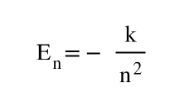🤓 Based on our data, we think this question is relevant for Professor Mieszawska's class at BROOKLYN CUNY.

# Solution: Using the Bohr model, determine the energy, in joules, necessary to ionize a ground-state hydrogen atom. Show your calculations.

###### Problem

Using the Bohr model, determine the energy, in joules, necessary to ionize a ground-state hydrogen atom. Show your calculations.

###### Solution

The equation of energy from Bohr model states that:where En is the energy at nth level, (2.179x10-18J) is a constant and n is the number of shellView Complete Written Solution